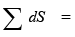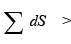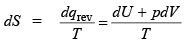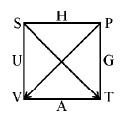Courses

# Condition Of Equilibrium And Criterion For A Spontaneous Process - Thermochemistry Chemistry Notes | EduRev

## Chemistry : Condition Of Equilibrium And Criterion For A Spontaneous Process - Thermochemistry Chemistry Notes | EduRev

The document Condition Of Equilibrium And Criterion For A Spontaneous Process - Thermochemistry Chemistry Notes | EduRev is a part of the Chemistry Course Physical Chemistry.
All you need of Chemistry at this link: Chemistry

Condition of equilibrium and criterion for a spontaneous process.

The entropy of a system remains unchanged in a reversible change while it increases in an irreversible change i.e.0  for reversible change
and0 for irreversible change

For a system with its surrounding

dsystem + dSsurrounding = 0 for reversible change
and                dsystem + dSsurrounding > 0 for irreversible change

then we have

dsystem + dSsurrounding ≥ 0

The criteria for reversible and irreversible process in terms. Of entropy as well as in terms U, H, A & G.

By the equation,We know that

qirr < qrev then
TdS > dqrev (for irreversible process)
Thus we write,       TdS = dU + PdV (for reversible process)
TdS > dU + PdV (for irreversible process)
Combining we get, TdS ≥ dU + PdV

(i)  Criterion in terms of change of entropy: Let U & V remains constant.
T(dS)U, V ≥ 0  or  (dS)U, V ≥ 0

The equal sign refers to reversible and greater-sign refers to an irreversible process.

(ii)  Criterion in terms of change of internal energy: Let S & V constant then

(dS)U, V ≥ 0

(iii) Criterion in terms of change of enthalpy: Let S & P are constant then

TdS ≥ dU + PdV
⇒         dU + PdV ≤ 0
But      dU + PdV = dH
⇒        (dH)S, P ≤ 0

(iv)  Criterion in terms of change of work function:

We know that              dA = dU – TdS & TdS ≥ dU + PdV

then  we get                dA ≤ –PdV

at constant volume and temperature (dA)V, T ≤ 0

(v)  Criterion in terms of change in free energy:

We know that       G = H – TS
⇒                         G = (U + PV) – TS
⇒                         dG = dU + PdV + VdP – TdS – SdT
&                          TdS ≥ dU + PdV, we get
⇒                         dG ≤ VdP – SdT

Let P & T are constant then

(dG)P, T ≤ 0

Using thermodynamic magic square we can find these criterion(dU)S, V ≤ 0,
(dH)S, P ≤ 0 (dG)P, T ≤ 0,
(dA)V, T ≤ 0 (dS)U, V ≥ 0

Offer running on EduRev: Apply code STAYHOME200 to get INR 200 off on our premium plan EduRev Infinity!

## Physical Chemistry

66 videos|94 docs|32 tests

,

,

,

,

,

,

,

,

,

,

,

,

,

,

,

,

,

,

,

,

,

;